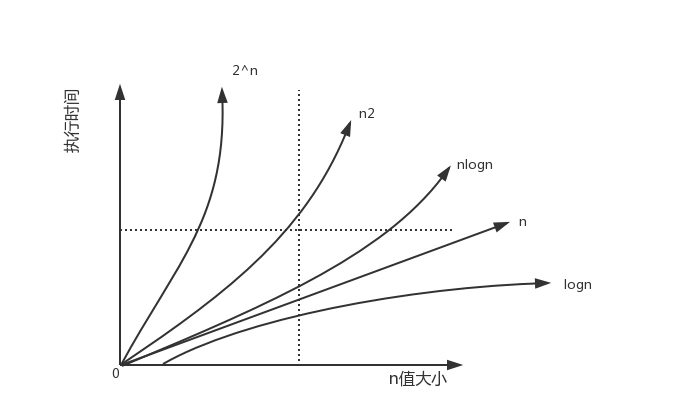# 通过js示例讲解时间复杂度与空间复杂度

1. 博客背景

2. 复杂度的表示方式

```T(n) = O(f(n))
S(n) = O(f(n)) ```

S表示的算法需要的总空间

f(n)表示的是代码执行的总次数

```function go(n) {
var item = 0;   // 这里执行了一次
for (var i = 0; i < n; i++) {  //这里执行了N次
for (var j = 0; j < n; j++) {   //这里执行了n*n次
item = item + i + j;   //这里执行了n*n次
}
}
return item; //这里执行了一次
}```

3. 时间复杂度

3.1 时间复杂度的定义

3.2 几种常见的时间复杂度

```for (var i = 0; i < n; i++) {
sum += i;
}```

```function total(n) {

console.log(1)
}```

T(n) = O(n²)

```function go(i) {
var sum = 0;
for (var j = 0; j < i; j++) {
sum += i;
}
return sum;
}
function main(n) {
var res = 0;
for (var i = 0; i < n; i++) {
res = res + go(i); // 这里是重点
}
}```

go：（1+n）

3.3 多块代码的时间复杂度

```    function go(n) {

for (var i = 0; i < n; i++) {
for (var j = 0; j < n; j++) {
console.log(1)
}
}

for (var i = 0; i < n; i++) {
console.log(2)
}
}```

3.4 对数阶和相加情况

```var i = 1;
while (i <= n) {
i = i * 10;
}```

i=logn

```function go(m,n) {

for (var i = 0; i < n; i++) {
console.log(1)
}

for (var i = 0; i < m; i++) {
console.log(2)
}

}```

4. 空间复杂度

4.1 空间复杂度的定义

4.2 常见的空间复杂度

• O(1)
```let a = 1;
let b = 1;
let c = 1;
let d = 1;```

• O(n)
`let arr =Array(n)`

O(n)

• O(n²)
```let arr=[]
for (var i = 0; i < n; i++) {
arr[i]=i
for (var j = 0; j < n; j++) {
arr[i][j]=j
}
}``````console.time('a')
function go(n) {
var item = 0;
for (var i = 1; i <= n; i++) {
item += i;
}
return item;
}
console.timeEnd('a')

console.time('b')
function go2(n) {
var item = n*(n+1)/2
return item;
}
console.timeEnd('b')

go(1000)
go2(1000)```

```function Fibonacci(n) {
if (n <= 1 ) {
return n;
} else {
return Fibonacci(n - 1) + Fibonacci(n - 2);
}
}

console.time('b')
Fibonacci(????)
console.timeEnd('b')```

JavaScript/Ajax教程-通过js示例讲解时间复杂度与空间复杂度